WEBINAR
Efficient Green Energy: Exploring Simulation-Driven Design for High-Torque Wind Turbine Machine
Thursday, September 21, 2023
Time
SESSION 1
SESSION 2
CEST (GMT +2)
03:00 PM
08:00 PM
EDT (GMT -4)
09:00 AM
02:00 PM
HOME / Applications / Parametric Analysis: Electrothermal Simulation of a DC Actuator using EMS inside SOLIDWORKS

# Parametric Analysis: Electrothermal Simulation of a DC Actuator using EMS inside SOLIDWORKS

Used Tools:## Problem description

The heat generated by the coil is one of the major design aspects of a DC linear actuator. In addition to significantly shortening the life expectancy of its mechanical components, the temperature changes can affect the actuator’s efficiency and repeatability. Hence, in this application note, an electrothermal simulation is performed versus multiple variables such as the coil thickness and input currents to study the heat distribution and variation at different conditions and design scenarios. Temperature-dependent material properties will be used at the end of this work to estimate the temperature variation of the actuator versus time while the electrical resistivity of the coil material changes.

Figure 1 shows the original DC linear actuator. Figure 2 contains the simulated 3D CAD model. Some non-magnetic parts were removed because they do not have any impact on the magnetic results like magnetic fields and force. However, they can affect thermal results but this effect will be neglected in this analysis. Moreover, the effect of a spring can be included in the motion study when the magnetic study is coupled to motion study.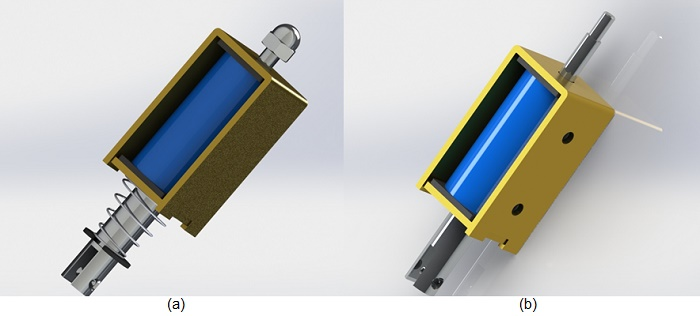Figure 1 - 3D CAD model of the DC actuator, a) original model, b) simulated model

Figure 2 illustrates the different components of the simulated DC actuator. The moving plunger and the actuator core are made of 12L14 carbon steel while the coil bobbin is made of an insulator material (FEP).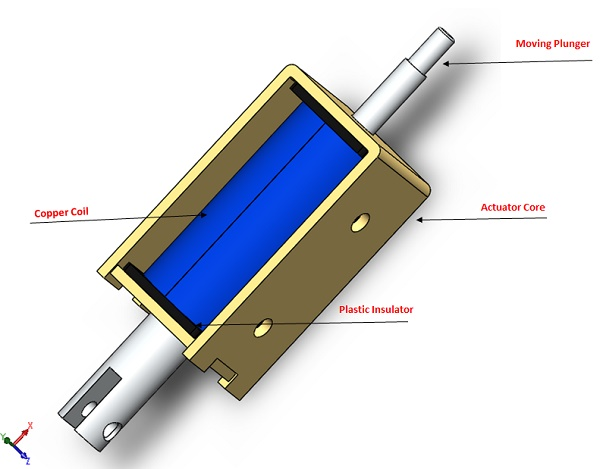Figure 2 - Different components of the simulated DC actuator

## Simulation & Results

### 1- Variable Plunger position

In magnetic static simulation, the heat is only produced by Joule Effect inside a conductor supplied with current. It is the only component where ohmic losses are generated.

The Ohmic loss or winding loss is expressed by the following relation:Where is the electrical resistivity of the coil material and is the current density of the coil.

Therefore, both the Ohmic loss and consequently the coil temperature are directly proportional to the coil material and the current density. Since the material of the coil is maintained the same, the current density will be varied. In this section, the input current is constant, and the coil dimensions will be changed to affect the current density results.

The coil current density depends mainly on the cross-section surface of the coil. Thus, the parametrized variable is the coil thickness as shown in Figure 3.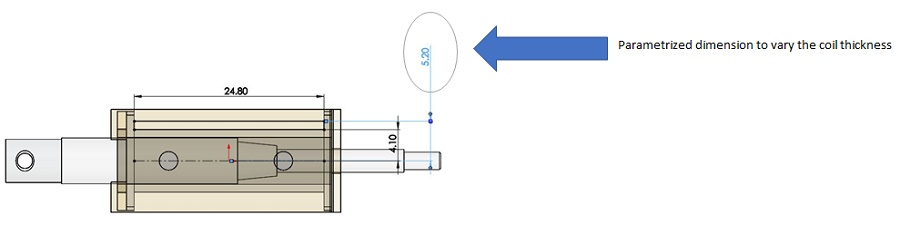Figure 3 - Parametrized dimension

Figures 4a) and 4b) illustrate, respectively, full, and cross section views of the magnetic field distribution when the coil thickness is 2.2mm. It is the maximum thickness allowed by the free space of the designed actuator. The magnetic field is around 0.55T in the actuator core while it reached 2T in the moving plunger.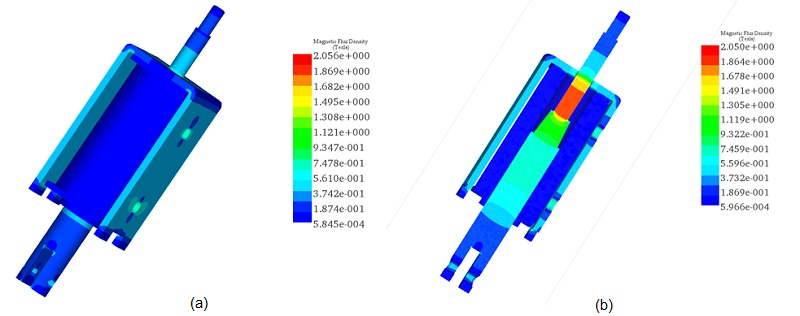Figure 4 - Magnetic flux density at a coil thickness of 2.2mm, a) full model view, b) cross section view

Figures 5a) and 5b) contain, respectively, full, and cross section views of the magnetic field distribution when the coil thickness is 1.1mm. The magnetic field results are the same as the previous scenario because input current is constant. A vector plot of the magnetic flux is shown in Figure 6.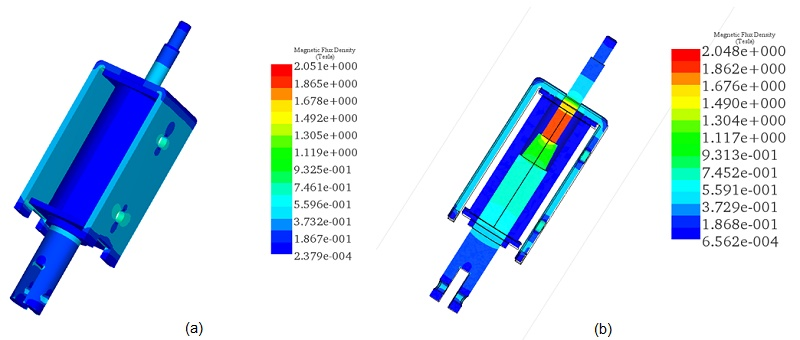Figure 5 - Magnetic flux density at a coil thickness of 1.1mm, a) full model view, b) cross section view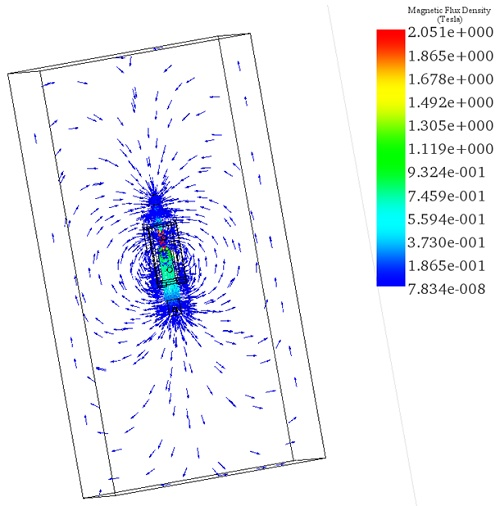Figure 6 - Cross section view of the magnetic field vector plot

The current density results versus coil thickness plots are shown in Figure 7. The current density is inversely proportional to the coil thickness. It reached a maximum value of 1.31e+7 Amp/m^2 when the thickness is 1.1mm and a minimum value of 6.59e+6 Amp/m^2 when the thickness is 2.2mm.The Ohmic losses results versus the coil thickness are plotted in Figure 8. At the thickness of 1.1mm, the coil Ohmic loss is 2.65W. Then, it decreases by increasing the coil thickness, i.e the coil volume increases. The minimal value is 1.34W measure at 2.2mm as thickness. The temperature evolution versus coil thickness should follow these behaviors.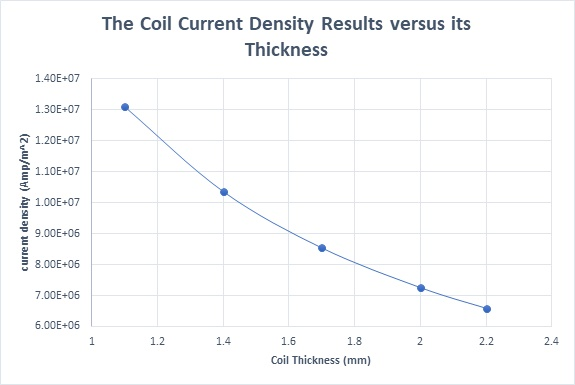Figure 7 - The coil current density results versus its thickness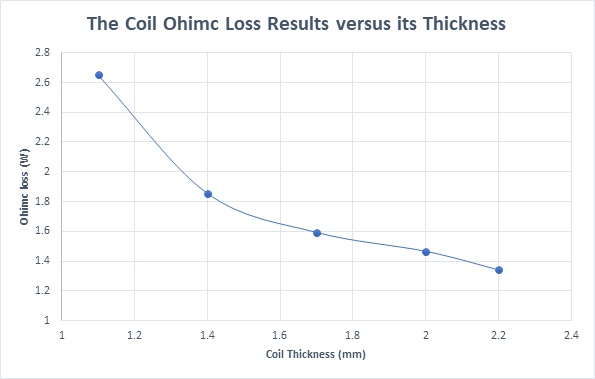Figure 8 - The coil Ohmic loss versus its thickness

Figure below contains the coil resistance variation versus its thickness. The resistance increases linearly from 0.094Ohm at 1.1mm to 0.106Ohm at 2.2mm.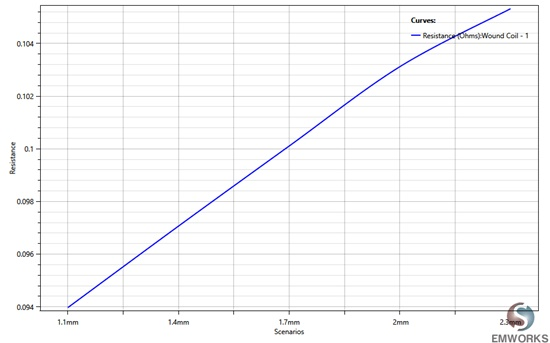Figure 9 - DC resistance of the coil versus thickness

Figures 10a) and 10b) demonstrate the steady state temperature distribution of the actuator, at the thickness of 1.1mm and 2.2mm, respectively. The computed temperature when the coil thickness is 1.1mm is 132 C while it is around 80 C in case of 2.2mm thickness. The maximum temperature is obtained in the source of heat in the actuator which is the coil and it has lower values in the other components which are in contact with the coil. Moreover, when the coil thickness becomes larger, more heat is propagated to the whole model as illustrated in Figure 10b). Figure 11 contains the temperature variation results versus different thicknesses of the coil. It follows the same behaviour as the current density variation.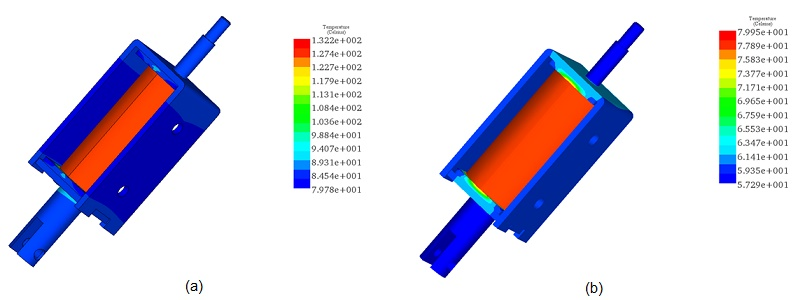Figure 10 - The actuator temperature results, a) with coil thickness of 1.1mm, b) with coil thickness of 2.2mm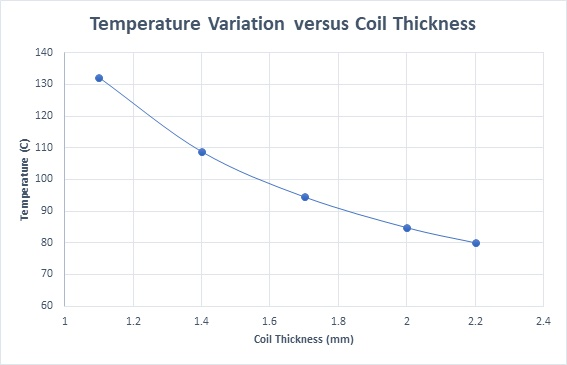Figure 11 - Temperature variation versus the coil thickness

### 2- Temperature Variation versus Coil current

In this section, the coil thickness is maintained constant at 2.2mm and the input current is varied. Values from 1A to 5A with step of 0.35A were used for the simulation.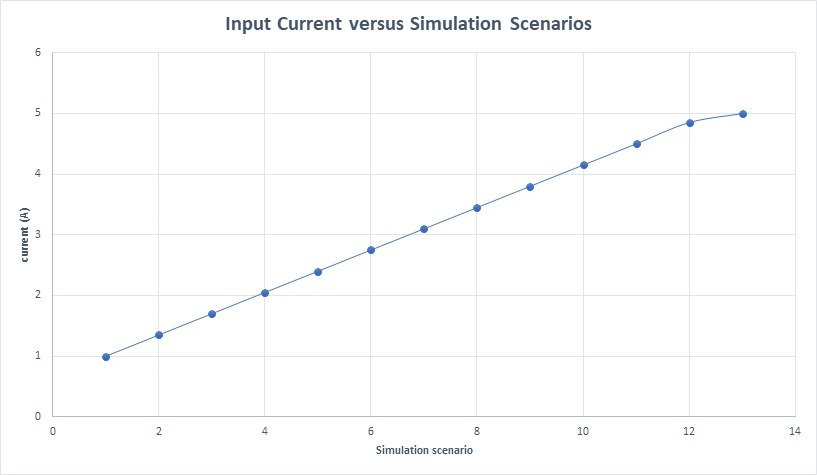Figure 12 - Input current versus simulation scenarios

Results of the magnetic flux density at current of 1A are given in Figures 13a) and 13b). The maximum reached magnetic field is around 1.29T in the moving plunger. At an input current of 5A, the magnetic field goes to peak value of 2.15T as shown in Figures 14a) and 14b).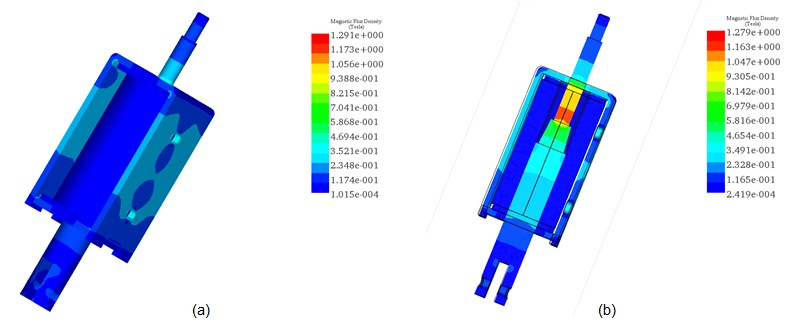Figure 13 - Magnetic flux distribution at 1A, a) full model, b) cross section view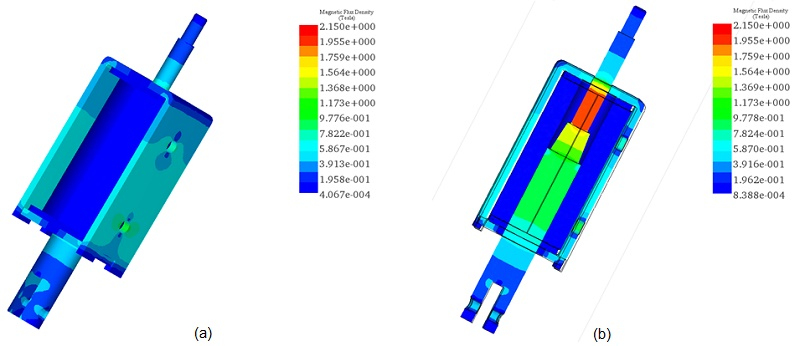Figure 14 - Magnetic flux distribution at 5A, a) full model, b) cross section view

The current density results calculated versus different input current rates is demonstrated in Figure 15. It represents a linear shape regarding the input current. Ohmic losses versus the coil currents are plotted in Figure 16.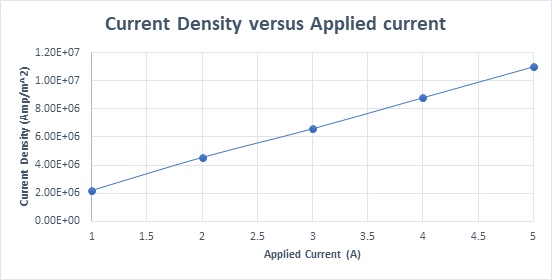Figure 15 - Current density versus applied current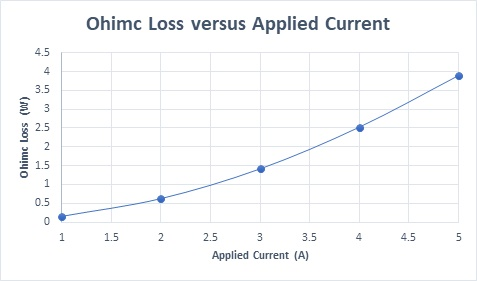Figure 16 - Ohmic loss versus input current

The cross-section plots in Figures 17a) and 17b), illustrate steady state temperature results, respectively, at 1A and 5A for the applied current. The predicted temperature of the actuator ranges from 30 C to 32 C at 1A while it varies from 110 C up to 174 C at 5A. The highest actuator temperature is evaluated in the coil in both cases. It propagates from the coil to the whole actuator bodies until it achieves the thermal equilibrium.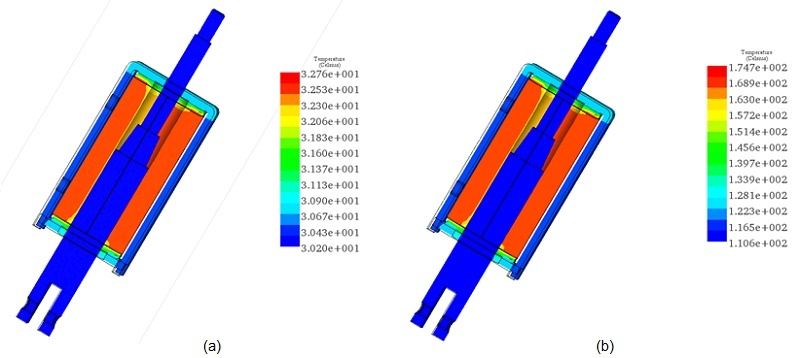Figure 17 - Cross section view of the temperature distribution, a) at 1A, b) at 5A

Figure 18 shows the maximum temperature in the actuator versus the different applied current rates. The temperature rises with the increase of the input current as explained above.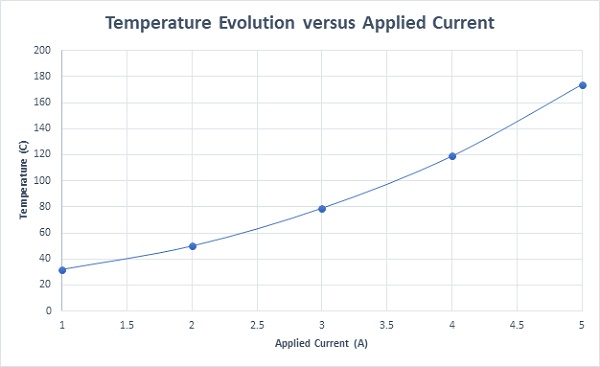Figure 18 - Temperature evolution versus applied current

## Thermal Analysis in case of temperature-dependent material property

In this final section, the copper coil is defined with temperature-depend electrical resistivity. The electrical conductivity of the copper versus temperature data is shown in Figure 19. This figure shows that the electrical conductivity of the copper decreases with the temperature i.e the electrical resistivity increases. Hence, when the temperature rises from its ambient value, the electrical resistivity of the copper winding will augment and consequently the Ohmic loss will increase also. Figure 20 illustrates the evolution of the coil Ohmic losses versus both temperature and time. Both curves confirm the previous statement. The coil copper loss increases linearly versus time to reach 2.33W after half of hour. After that, the loss becomes almost constant which indicates that the steady state is reached.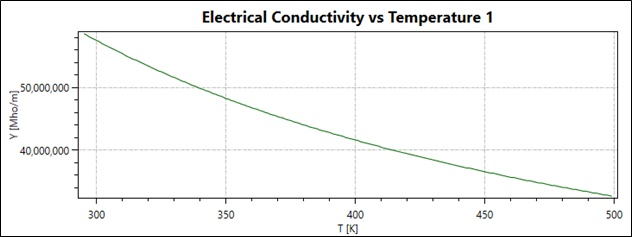Figure 19 - The copper electrical conductivity versus temperature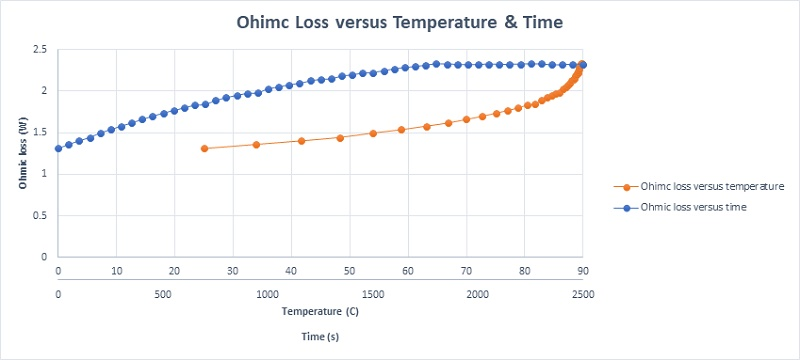Figure 20 - The coil Ohmic losses versus both temperature and time

Since the coil material changes with temperature, thus its resistance will be affected. Figure 21 gives a plot of the coil DC resistance versus both time and temperature. It shows that the DC resistance increases with temperature. It measures a value of 0.1035Ohm at the ambient temperature and achieves its steady state value of 0.1295Ohm after a half of hour.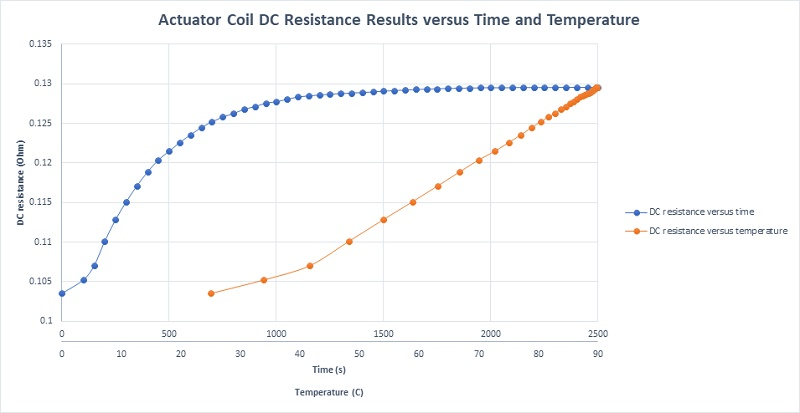Figure 21 - RD resistance of the coil actuator versus both temperature and time

Figure 22 contains the temperature variation of the coil. It mimics the Ohmic loss evolution. The temperature increases from 25 C at t=0 to the steady state temperature of 90.03 C at t=1800 s. The actuator steady state temperature distribution is plotted in Figure 23.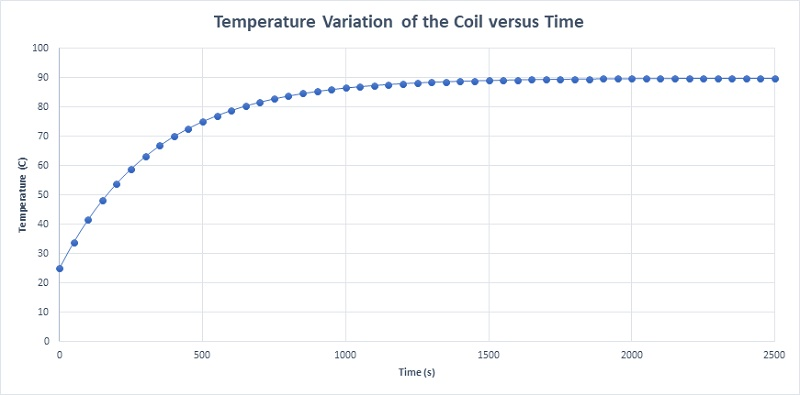Figure 22 - Temperature evolution of the coil versus time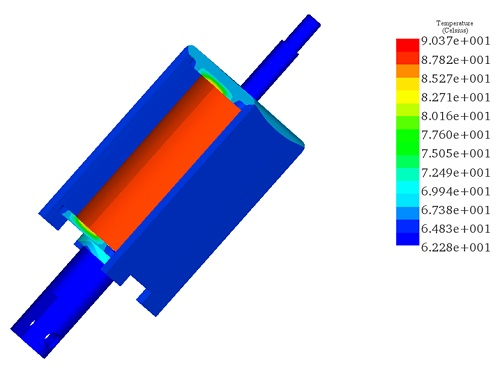Figure 23 -Temperature distribution of the actuator after half hour

## Summary

In this application, EMS was used to study a linear DC actuator. The temperature results were under different operating conditions like using different coil thicknesses and input currents. The coil material was varied to make the simulation more realistic by using temperature depend electrical resistivity. The goal behind these simulation is to help users to reduce the heat generated by a coil in a DC actuator which can improve its reliability and efficiency.